# Test: Source Transformations

## 10 Questions MCQ Test Network Theory (Electric Circuits) | Test: Source Transformations

Description
Attempt Test: Source Transformations | 10 questions in 10 minutes | Mock test for Electrical Engineering (EE) preparation | Free important questions MCQ to study Network Theory (Electric Circuits) for Electrical Engineering (EE) Exam | Download free PDF with solutions
QUESTION: 1

### A voltage source connected in series with a resistor can be converted to a?

Solution:

A voltage source connected in series can be converted to a current source connected in parallel using the relation obtained from ohm’s law, that is V=IR. This equation shows that a voltage source connected in series has the same impact as a current source connected in parallel.

QUESTION: 2

### Calculate the total current in the circuit.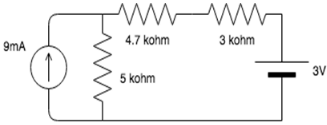Solution:

The 9mA source connected in parallel to the 5 kohm resistor can be converted to a 45V source in series with a 5 kohm resistor. Applying mesh analysis, we get:
I=(45-3)/(5+4.7+3)= 3.3mA.

QUESTION: 3

### Find the value of voltage once source transformation is applied to the circuit.​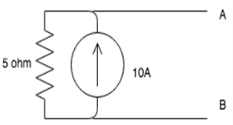Solution:

Using ohm’s law, we can use the relation: V=IR.
Thus V=10*5= 50V.

QUESTION: 4

Once the circuit is transformed to a voltage source where will the resistance be connected?​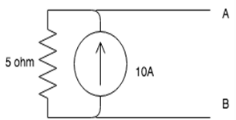Solution:

The resistance is connected in series with the voltage source because we are transforming a current source connected in parallel to a resistor to a voltage source connected in series with it.

QUESTION: 5

What will the value of the current be once source transformation is applied to the circuit?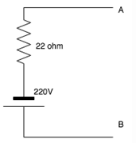Solution:

Using ohm’s law, we can use the relation: V=IR.
Thus I=V/R.
I=220/22=10A.

QUESTION: 6

Once the circuit is transformed to a current source where will the resistance be connected?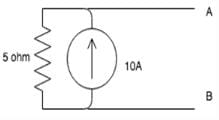Solution:

The resistance is connected in series with the voltage source because we are transforming a current source connected in parallel to a resistor to a voltage source connected in series with it.

QUESTION: 7

A current source connected in parallel with a resistor can be converted to a?

Solution:

A current source connected in parallel can be converted to a voltage source connected in series using the relation obtained from ohm’s law, that is V=IR. This equation shows that a current source connected in parallel has the same impact as a voltage source connected in series.

QUESTION: 8

A source transformation is_________

Solution:

A source transformation is bilateral because a voltage source can be converted to a current source and vice-versa.

QUESTION: 9

In source transformation________

Solution:

In source transformation, the value of the voltage and current sources change when changed from voltage to current source and current to voltage source but the value of the resistances remains the same.

QUESTION: 10

If there are 3 10V sources connected in parallel then on source transformation__________

Solution:

When voltages are connected in parallel, the effect of only one source is considered because the effect of the voltage remains the same when connected in parallel.Use Code STAYHOME200 and get INR 200 additional OFF Use Coupon Code